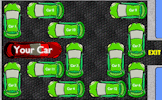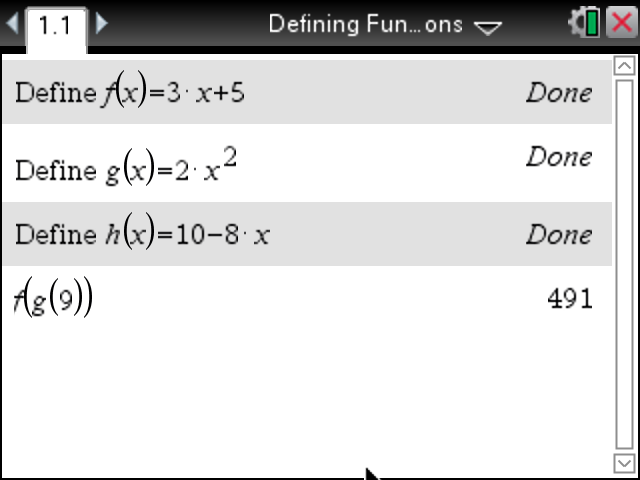# Functions

## An online exercise on function notation, inverse functions and composite functions.

##### Level 1Level 2Level 3Level 4Level 5Level 6Exam-StyleDescriptionHelpMore Algebra

This is level 1, describe function machines using function notation. You can earn a trophy if you get at least 9 correct and you do this activity online. The first question has been done for you.

 $$x \to$$$$\times 3$$$$\to$$$$+ 4$$$$\to$$$$x \to$$$$\times 5$$$$\to$$$$+ 1$$$$\to$$$$x \to$$$$\times 2$$$$\to$$$$- 7$$$$\to$$$$x \to$$$$- 6$$$$\to$$$$\times 4$$$$\to$$$$x \to$$$$+ 3$$$$\to$$$$\times 6$$$$\to$$$$x \to$$$$+ 9$$$$\to$$$$\times 3$$$$\to$$$$x \to$$$$\times 2$$$$\to$$$$+ 5$$$$\to$$$$x \to$$$$\times 5$$$$\to$$$$- 3$$$$\to$$$$x \to$$$$- 8$$$$\to$$$$\times 13$$$$\to$$$$x \to$$$$+ 16$$$$\to$$$$\times 20$$$$\to$$$$x \to$$$$+ 14$$$$\to$$$$\times 25$$$$\to$$$$x \to$$$$\times 43$$$$\to$$$$- 5.5$$$$\to$$Check

This is Functions level 1. You can also try:
Level 2 Level 3 Level 4 Level 5 Level 6

## Instructions

Try your best to answer the questions above. Type your answers into the boxes provided leaving no spaces. As you work through the exercise regularly click the "check" button. If you have any wrong answers, do your best to do corrections but if there is anything you don't understand, please ask your teacher for help.

When you have got all of the questions correct you may want to print out this page and paste it into your exercise book. If you keep your work in an ePortfolio you could take a screen shot of your answers and paste that into your Maths file.

## Transum.org

This web site contains over a thousand free mathematical activities for teachers and pupils. Click here to go to the main page which links to all of the resources available.## More Activities:

Mathematicians are not the people who find Maths easy; they are the people who enjoy how mystifying, puzzling and hard it is. Are you a mathematician?

Comment recorded on the 5 April 'Starter of the Day' page by Mr Stoner, St George's College of Technology:

"This resource has made a great deal of difference to the standard of starters for all of our lessons. Thank you for being so creative and imaginative."

Comment recorded on the 9 April 'Starter of the Day' page by Jan, South Canterbury:

"Thank you for sharing such a great resource. I was about to try and get together a bank of starters but time is always required elsewhere, so thank you."

Each month a newsletter is published containing details of the new additions to the Transum website and a new puzzle of the month.

The newsletter is then duplicated as a podcast which is available on the major delivery networks. You can listen to the podcast while you are commuting, exercising or relaxing.

Transum breaking news is available on Twitter @Transum and if that's not enough there is also a Transum Facebook page.

#### Car Park PuzzleCan you get your car out of the very crowded car park by moving other cars forwards or backwards? There are five levels of increasing difficulty and the interactive interface makes this a fun problem solving exercise.

There are answers to this exercise but they are available in this space to teachers, tutors and parents who have logged in to their Transum subscription on this computer.

A Transum subscription unlocks the answers to the online exercises, quizzes and puzzles. It also provides the teacher with access to quality external links on each of the Transum Topic pages and the facility to add to the collection themselves.

Subscribers can manage class lists, lesson plans and assessment data in the Class Admin application and have access to reports of the Transum Trophies earned by class members.

Subscribe

## Go Maths

Learning and understanding Mathematics, at every level, requires learner engagement. Mathematics is not a spectator sport. Sometimes traditional teaching fails to actively involve students. One way to address the problem is through the use of interactive activities and this web site provides many of those. The Go Maths page is an alphabetical list of free activities designed for students in Secondary/High school.

## Maths Map

Are you looking for something specific? An exercise to supplement the topic you are studying at school at the moment perhaps. Navigate using our Maths Map to find exercises, puzzles and Maths lesson starters grouped by topic.

## Teachers

If you found this activity useful don't forget to record it in your scheme of work or learning management system. The short URL, ready to be copied and pasted, is as follows:

Alternatively, if you use Google Classroom, all you have to do is click on the green icon below in order to add this activity to one of your classes.

It may be worth remembering that if Transum.org should go offline for whatever reason, there are mirror sites at Transum.com and Transum.info that contain most of the resources that are available here on Transum.org.

When planning to use technology in your lesson always have a plan B!

Inverse Joke, Anon

Tuesday, June 16, 2020

"I poured root beer into a square glass. Now I just have beer!"

Do you have any comments? It is always useful to receive feedback and helps make this free resource even more useful for those learning Mathematics anywhere in the world. Click here to enter your comments.For All:

© Transum Mathematics :: This activity can be found online at:
www.transum.org/Maths/Exercise/Functions.asp?

## Description of Levels

CloseLevel 1 - Describe function machines using function notation.

Level 2 - Evaluate the given functions.

Level 3 - Solve the equations given in function notation.

Level 4 - Find the inverse of the given functions.

Level 5 - Simplify the composite functions.

Level 6 - Mixed questions.

Exam Style questions are in the style of GCSE or IB/A-level exam paper questions and worked solutions are available for Transum subscribers.

Answers to this exercise are available lower down this page when you are logged in to your Transum account. If you don’t yet have a Transum subscription one can be very quickly set up if you are a teacher, tutor or parent.

## Functions

The following notes are intended to be a reminder or revision of the concepts and are not intended to be a substitute for a teacher or good textbook.

### Level 1: Describe function machines using function notation.

Function notation is quite different to the algebraic notation you have learnt involving brackets. $$f(x)$$ does not mean the value of f multiplied by the value of x. In this case f is the name of the function and you would read $$f(x) = x^2$$ as "f of x equals x squared".

In terms of function machines, if the input is $$x$$ then the output is $$f(x)$$.

Example

$$x \to$$$$+ 3$$$$\to$$$$\times 4$$$$\to f(x)$$

In this case 3 is added to $$x$$ and then the result is multiplied by 4 to give $$f(x)$$

$$(x+3) \times 4 = f(x)$$

$$f(x) = 4(x+3)$$

### Level 2: Evaluate the given functions.

Example

if $$f(x)=x^2 + 3$$ calculate the value of $$f(6)$$

This means replace the $$x$$ with a 6 in the given function to obtain the result.

$$f(6) = 6^2+3$$

$$f(6) = 39$$

### Level 3: Solve the equations given in function notation.

Example

$$f(x)=3(x+7)$$ find $$x$$ if $$f(x) = 30$$

$$3(x+7)=30$$

$$x+7 = 10$$

$$x = 3$$

### Level 4: Find the inverse of the given functions.

The inverse of a function, written as $$f^{-1}(x)$$ can be thought of as a way to 'undo' the function. If the function is written as a function machine, the inverse can be thought of as working backwards with the output becomming the input and the input becoming the output.

Example

$$f(x) = 4(x+3)$$

$$x \to$$$$+ 3$$$$\to$$$$\times 4$$$$\to f(x)$$

$$f^{-1}(x) \leftarrow$$$$- 3$$$$\leftarrow$$$$\div 4$$$$\leftarrow x$$

$$f^{-1}(x) = \frac{x}{4} - 3$$

A quicker way of finding the inverse of $$f(x)$$ is to replace the $$f(x)$$ with $$x$$ on the left side of the equals sign and replace the $$x$$ with $$f^{-1}(x)$$ on the right side of the equals sign. Then rearrange the equation to make $$f^{-1}(x)$$ the subject.

### Level 5: Simplify the composite functions.

A composite function contains two functions combined into a single function. One function is applied to the result of the other function. You should evaluate the function closest to $$x$$ first.

Example

if $$f(x)=2x+7$$ and $$g(x)=5x^2$$ find $$fg(3)$$

$$g(3) = 5 \times 3^2$$

$$g(3) = 5 \times 9$$

$$g(3) = 45$$

$$f(45) = 2 \times 45 + 7$$

$$f(45) = 97$$

so $$fg(3) = 97$$

Example

if $$f(x)=x+2$$ and $$g(x)=3x^2$$ find $$gf(x)$$

$$gf(x) = 3(x+2)^2$$

$$gf(x) = 3(x^2+4x+4)$$

$$gf(x) = 3x^2+12x+12$$

### Level 6: Mixed questions.

Example

Find $$f(x-2)$$ if $$f(x)=5x^2+3$$

$$f(x-2) =5(x-2)^2+3$$

$$f(x-2) =5(x^2-4x+4)+3$$

$$f(x-2) =5x^2-20x+20+3$$

$$f(x-2) =5x^2-20x+23$$

Did you know some calculators can apply functions?

TI-nSpire:Don't wait until you have finished the exercise before you click on the 'Check' button. Click it often as you work through the questions to see if you are answering them correctly. You can double-click the 'Check' button to make it float at the bottom of your screen.

Answers to this exercise are available lower down this page when you are logged in to your Transum account. If you don’t yet have a Transum subscription one can be very quickly set up if you are a teacher, tutor or parent.

Close